#### 期刊菜单

Source-Storage Coordinated Optimal Scheduling Method Based on Energy Storage Participating in Regulation
DOI: 10.12677/SG.2021.116038, PDF, HTML, XML, 下载: 171  浏览: 269  科研立项经费支持

Abstract: In the background of China’s “dual-carbon” target development strategy, the development of new energy, mainly wind power and photovoltaics, is accelerating. This has brought great challenges to the scheduling and operation of the power grid, and abandoning wind and light has become an urgent problem to be solved. At the same time, the rapid development of large-capacity energy storage in recent years provides a new way for new energy consumption with excellent charge/ discharge adjustment characteristics and adjustment capabilities. To this end, this paper proposes a source-storage coordinated optimal scheduling method based on improving the capacity of new energy consumption. Firstly, the influence mechanism of source-storage coordinated scheduling on new energy consumption is analyzed. Then, the source-storage coordinated optimal scheduling model was established and solved with the goal of maximizing the consumption of new energy. Finally, a case analysis is carried out on the background of large-scale wind power photovoltaic integration into the power grid, which verifies the effectiveness of the source-storage coordinated optimal scheduling method proposed in this paper for the consumption of new energy.

1. 引言

2. 源–储协调运行对新能源消纳的影响机理

2.1. 风电光伏出力特性

2.1.1. 风电出力特性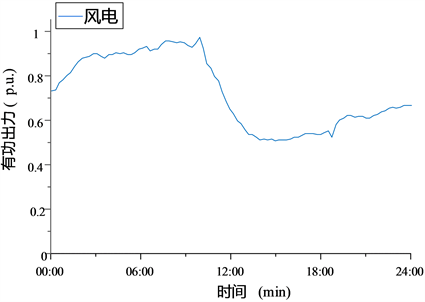Figure 1. Operating curve of a wind station in Gansu province on a certain day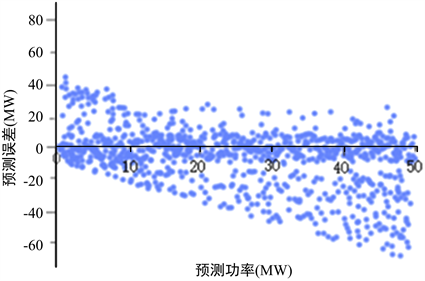Figure 2. Scatter diagram of power prediction error changing with predicted power of a wind station in Gansu

2.1.2. 光伏出力特性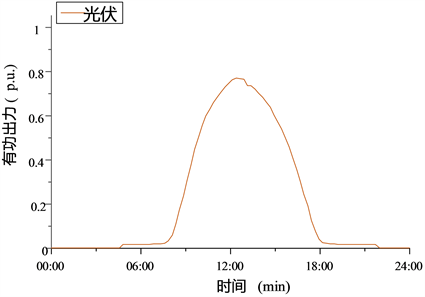Figure 3. The operating curve of a photovoltaic power station in Gansu province on a certain day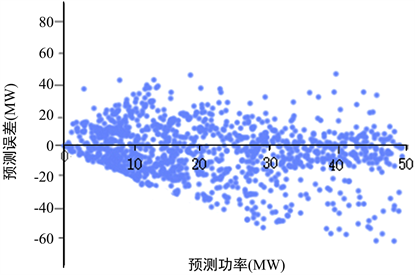Figure 4. Scatter diagram of power prediction error changing with predicted power of a photovoltaic power station in Gansu

2.2. 大容量储能电站调节特性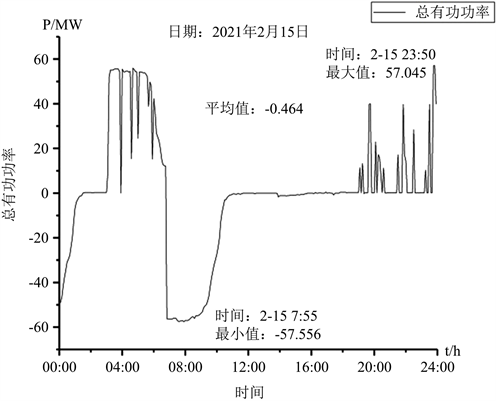Figure 5. Operating curve of Blongi energy storage power station in Gansu on August 12th

2.3. 源储协调调度对新能源消纳的影响机理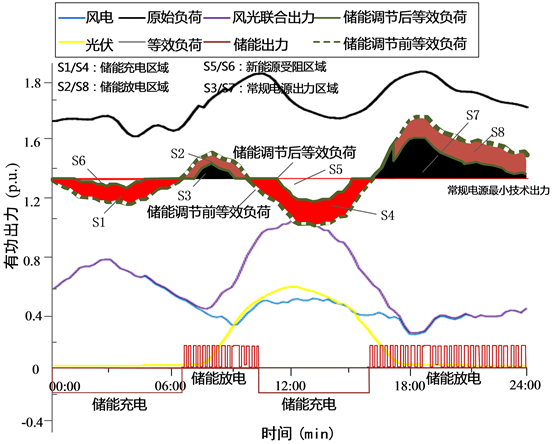Figure 6. Schematic diagram of impact of coordinated source-storage operation on new energy consumption

3. 基于储能参与调节的源–储协调优化调度方法

3.1. 蒙特卡洛模拟与场景削减

3.1.1. 蒙特卡洛模拟法

$\stackrel{¯}{X}=\underset{i=1}{\overset{k}{\sum }}{X}_{i}/k$ (1)

3.1.2. 场景削减

${d}_{ij}=\sqrt{\underset{k=1}{\overset{N}{\sum }}{\left({Y}_{ki}-{Y}_{kj}\right)}^{2}}$ (2)

3.2. 目标函数

$\mathrm{max}W=\underset{i=1}{\overset{{T}_{i}}{\sum }}\left(\underset{s=1}{\overset{K}{\sum }}\left({\pi }_{s}\underset{j=1}{\overset{{N}_{W}}{\sum }}{P}_{W,j}^{t}+{\pi }_{s}\underset{j=1}{\overset{{N}_{PV}}{\sum }}{P}_{PV,j}^{t}\right)\right)\Delta t$ (3)

3.3. 约束条件

1) 系统功率平衡约束

$\underset{j=1}{\overset{{N}_{PV}}{\sum }}{P}_{PV,j}^{t}+\underset{j=1}{\overset{{N}_{w}}{\sum }}{P}_{W,j}^{t}+\underset{j=1}{\overset{{N}_{G}}{\sum }}{P}_{G,j}^{t}\text{+}\underset{j=1}{\overset{{N}_{HP}}{\sum }}H{P}_{j}^{t}\text{+}\underset{j=1}{\overset{{N}_{E}}{\sum }}{P}_{E,j}^{t}={P}_{L}^{t}$ (4)

2) 常规火电机组运行约束

a) 机组技术出力约束：

${U}_{Gj}^{t}{P}_{Gj,\mathrm{min}}\le {P}_{Gj}^{t}\le {U}_{Gj}^{t}{P}_{Gj,\mathrm{max}}$ (5)

b) 机组爬坡速率约束：

$\left\{\begin{array}{l}{U}_{Gj}^{t}{P}_{G,j}^{t}-{U}_{Gi}^{t-1}{P}_{G,j}^{t-1}\le {P}_{G,j,\text{up}}\\ {U}_{Gi}^{t-1}{P}_{G,j}^{t-1}-{U}_{G}^{t}{P}_{G,j}^{t}\le {P}_{G,j,\text{down}}\end{array}$ (6)

c) 机组最小启停时间约束：

$\left\{\begin{array}{l}\left({U}_{Gj}^{t-1}-{U}_{Gj}^{t}\right)\left({T}_{Gj,\text{on}}^{t}-{T}_{Gj,\text{on}}^{\mathrm{min}}\right)\ge 0\\ \left({U}_{Gj}^{t-1}-{U}_{Gj}^{t}\right)\left({T}_{Gj,\text{off}}^{t}-{T}_{Gj,\text{off}}^{\mathrm{min}}\right)\ge 0\end{array}$ (7)

3) 常规水电机组运行约束

a) 机组出力约束：

$H{P}_{j,\mathrm{min}}\le H{P}_{j}^{t}\le H{P}_{j,\mathrm{max}}$ (8)

b) 爬坡速率约束：

$\left\{\begin{array}{l}H{P}_{j}^{t}-H{P}_{j}^{t-1}\le H{P}_{j,\text{up}}\\ H{P}_{j}^{t-1}-H{P}_{j}^{t}\le H{P}_{j,\text{down}}\end{array}$ (9)

4) 风电运行约束

$0\le {P}_{W,j}^{t}\le {P}_{W,F,j}^{t}$ (10)

5) 光伏运行约束

$0\le {P}_{PV,j}^{t}\le {P}_{PV,F,j}^{t}$ (11)

6) 储能电站运行约束

a) 储能最大充放电功率约束：

${P}_{E,j\mathrm{min}}\le {P}_{E,j}^{t}\le {P}_{E,j\mathrm{max}}$ (12)

b) 储能荷电状态约束：

$SO{C}_{\mathrm{min}}\le SOC\left(t\right)\le SO{C}_{\mathrm{max}}$ (13)

c) 储能充放电平衡约束：

$\underset{i=1}{\overset{{T}_{i}}{\sum }}{P}_{E,j}^{t}=0$ (14)

3.4. 模型求解

a) 输入DESO系统参数及算法参数。算法参数包括最大进化代数G、种群规模MP、个体维度D、缩放因子S和交叉概率CR。系统参数包括风电光伏日前预测数据、传统电源调节参数、储能电站调节性能参数等。

b) 种群初始化。生产初始化种群，种群中的每个个体代表一组控制变量，包括传统电源计划出力、风电场计划出力、光伏电站计划出力及储能日前充放电计划。

c) 计算适应度。计算种群中每个个体的适应度，选择最优适应度个体。

d) 处理约束条件。当个体不满足源–储协调优化调度模型的约束条件时，修改其适应度值将其淘汰。

e) 实施采用双变异策略的变异操作。计算种群相似度，并根据当前种群的相似度选择合适的变异策略。

f) 交叉和选择。进行种群交叉，从中选择出新一代种群。

g) 自适应调整交叉概率。对交叉概率按个体优异性进行自适应调整，将优异个体的交叉概率保留到下一代。

h) 重复步骤c~g，直到达到最大进化代数，输出储能日前充放电计划、传统电源日前出力计划、新能源日前出力计划，新能源消纳电量W。

4. 算例分析

4.1. 算例概述Table 1. Regulation parameters of conventional power sourcesTable 2. Parameters of lithium iron phosphate battery energy storage power station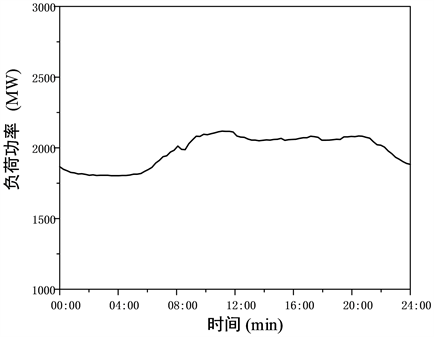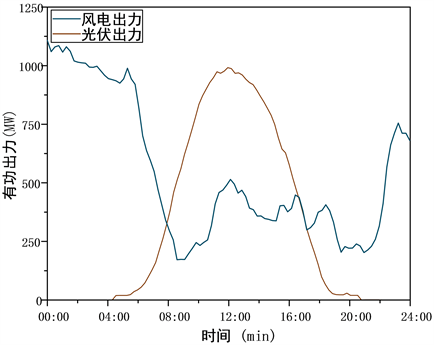Figure 8. Day-ahead forecast output of new energy

4.2. 算例分析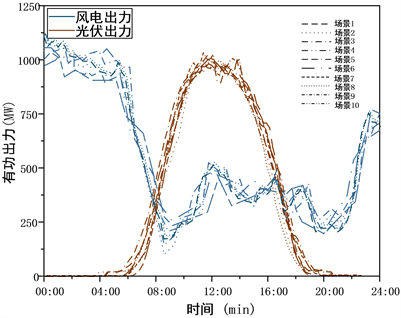Figure 9. Day-ahead forecast output of new energyTable 3. Typical scenario probability of day-ahead output prediction error of new energy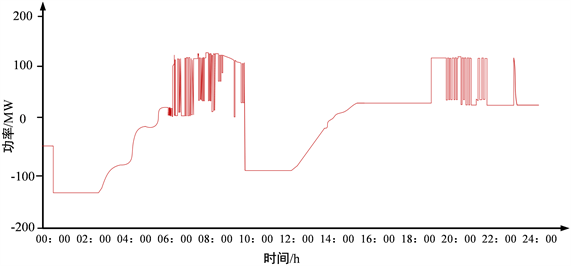Figure 10. Day-ahead charge and discharge plan of energy storage station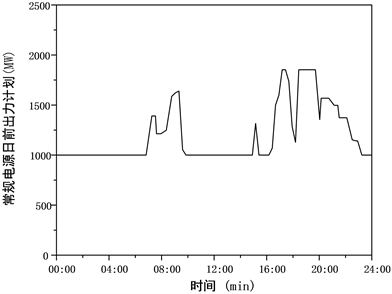Figure 11. Day-ahead output plan of conventional power sources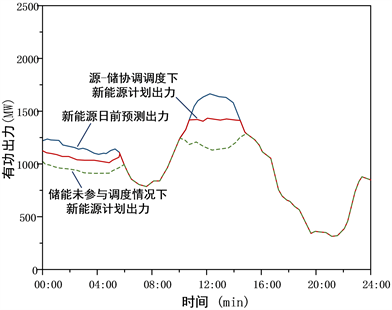Figure 12. The comparison of new energy output plans before and after source-storage coordinated schedulingTable 4. Comparison of new energy consumption before and after energy storage participating in coordinated scheduling

5. 结论

1) 大容量电池储能具有优良的充/放电特性和调节能力。首先，其[20%, 80%]的充放电深度为消纳新能源提供了有利条件。随着电池储能容量的扩大，其新能源消纳能力将进一步提高。其次，大容量电池储能的快速响应能力能有效应对风光出力的随机波动性，通过灵活调节充放电功率以平抑新能源出力随机波动性。

2) 将大容量电池储能纳入调度，实施源–储优化调度，可有效提高系统调峰能力。储能在新能源受阻时段充电，在新能源非受阻时段优先放电，随后常规电源弥补功率缺额出力。通过该源–储协调调度模式相比于储能不参与调度的模式，能够更有效地促进新能源消纳。

  李政, 陈思源, 董文娟, 刘培, 杜尔顺, 麻林巍, 何建坤. 碳约束条件下电力行业低碳转型路径研究[J]. 中国电机工程学报, 2021, 41(12): 3987-4001. https://kns.cnki.net/kcms/detail/detail.aspx?dbcode=CJFD&dbname=CJFDLAST2021&filename=ZGDC202112001&uniplatform=NZKPT&v=3UDY-INjhgp7gGHI5q7kjxrWxycnodJ9MuOcwE4D8sxhl9eTKG6B2NdtrvAGB-gk  Aneke, M. and Wang, M. (2016) Energy Storage Technologies and Real Life Applications—A State of the Art Review. Applied Energy, 179, 350-377. https://doi.org/10.1016/j.apenergy.2016.06.097  Amrouche, S.O., Rekioua, D., Rekioua, T., et al. (2016) Overview of Energy Storage in Renewable Energy Systems. International Journal of Hydrogen Energy, 41, 20914-20927. https://doi.org/10.1016/j.ijhydene.2016.06.243  金力, 房鑫炎, 蔡振华, 陈东海, 李亦凡. 考虑特性分布的储能电站接入的电网多时间尺度源储荷协调调度策略[J]. 电网技术, 2020, 44(10): 3641-3650.  程庭莉. 含分布式储能的主动配电网多目标优化调度方法研究[D]: [博士学位论文]. 重庆: 重庆大学, 2018.  崔杨, 周慧娟, 仲悟之, 赵钰婷, 崔成伟. .考虑火电调峰主动性与需求响应的含储能电力系统优化调度[J]. 高电压技术, 2021, 47(5): 1674-1684. https://kns.cnki.net/kcms/detail/detail.aspx?dbcode=CJFD&dbname=CJFDLAST2021&filename=GDYJ202105017&uniplatform=NZKPT&v=4wc-ki0R37SwiLi59aRleUt9mAAD-PuNE_h5grz_UVfN4dpfWFyErC86EHfWygNA  赵书强, 王扬, 徐岩, 殷加玞. 基于机会约束目标规划的高风电接入比例下大规模储能与火电协调调度[J]. 中国电机工程学报, 2016, 36(4): 969-977.  李军徽, 张嘉辉, 穆钢, 葛延峰, 严干贵, 史松杰. 储能辅助火电机组深度调峰的分层优化调度[J]. 电网技术, 2019, 43(11): 3961-3970.  赵书强, 刘大正, 谢宇琪, 等. 基于相关机会目标规划的风光储联合发电系统储能调度策略[J]. 电力系统自动化, 2015, 39(14): 30-36+53.  陈玉敏. 基于场景分析的源荷储多时间尺度协调调度研究[D]: [硕士学位论文]. 北京: 华北电力大学, 2020.  刘波, 王凌, 金以慧. 差分进化算法研究进展[J]. 控制与决策, 2007, 22(7): 721-729.  林少伯, 韩民晓, 赵国鹏, 等. 基于随机预测误差的分布式光伏配网储能系统容量配置方法[J]. 中国电机工程学报, 2013, 33(4): 25-33+前插4.  于东, 孙欣, 高丙团, 等. 考虑风电不确定出力的风电并网协调优化模型[J]. 电工技术学报, 2016, 31(9): 34-41.